Partial Fraction Decomposition All TypesPartial Fraction Decomposition All Types

Learn about the different types of partial fraction decomposition in this free math video tutorial by Mario’s Math Tutoring. We discuss linear factors, repeated linear factors, quadratic factors, repeated quadratic factors as well as improper fractions.

0:23 Linear Factors Example 1
4:14 Linear Factors Example 2
8:27 Repeated Linear Factors Example 1
11:07 Repeated Linear Factors Example 2
28:42 Improper Fractions

Looking to raise your math score on the ACT and new SAT?
Check out my Huge ACT Math Video Course and my Huge SAT Math Video Course for sale at
http://mariosmathtutoring.teachable.com

http://www.mariosmathtutoring.com

* Organized List of My Video Lessons to Help You Raise Your Scores & Pass Your Class. Videos Arranged by Math Subject as well as by Chapter/Topic. (Bookmark the Link Below)
http://www.mariosmathtutoring.com/free-math-videos.html

algebra tutors,partial fraction decomposition,how to do partial fraction,what are partial fractions,partial fractions,decomposition,fraction,calculus,improper fractions,repeated quadratic factors,partial,quadratic factors,factors,linear factors,expression,free,math,maths,mathematics,how to,video,tutorial,mario’s math tutoring,equating coefficients,rational function,break down,precalculus,fractions,repeated linear factors

algebratutors.org

20 Responses to “Partial Fraction Decomposition All Types”

1.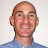Mario's Math Tutoring says:

Glad to see this continues to be a helpful video for students…If you want clarification on these different types check out the partial fraction playlist I put together here:

2.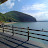koneh Norbert says:

thanks alot

3.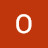Olamide Omikunle says:

Wow. U made me pass my assignment. 😁

4.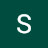Sanskruti Badhe says:

For 28:30 A=0, B=-2, C=0, D=1, E=3

5.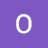OMWOYO MOSE JUSPER says:

i'm taking my control systems classes.i came here to remind myself because i'm studying on first order and second order systems

6.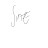aash syed says:

PLEASE DON'T STOP DOING WHAT YOU ARE DOING. THE FUTURE WORLD NEEDS YOU.

7.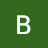209X1A0303 BONAGIRI SAIROOPA says:

very useful to inter and b tech students

8.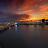lyric Cook says:

CRYSTAL CLEAR
Thank you

9.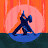DaSlothh says:

You deserve a medal.

10.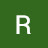Faisal Ruba says:

Where can I find the answer of the repeated quarderic factor?

11.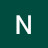Ndahambelela Tungembo says:

12.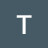Timothy Hutfles says:

Helped so much in my diff eq class!
Thanks so much man!

13.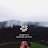Davis Rine says:

THANK YOU I GOT IT LIKE IN 5 MINUTES! THANKS ALOT

14.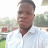Ssekamanya Arafat says:

Thanks though the camera distance I too far

15.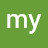my happiness says:

can you teach us how to solve differential and integration calculus ,i leaned a lot because of you please make a video of it

16.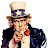Cruz Marco says:

Partial Fraction is the final boss of algebra, often used in higher math, worth mastering.

17.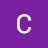Conor Walsh says:

Top man Mario

18.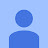Zen Wei Yap says:

A= 0,B = -2,C = 0,D=1,E=3

19.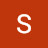Samuel Akalewold says:

excellent

20.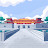jiyash_06 says:

im worried to see eastern country college student find it hard i got 100 percent in calculus at grade 5 i
m chinese who wanna compete in calculus?